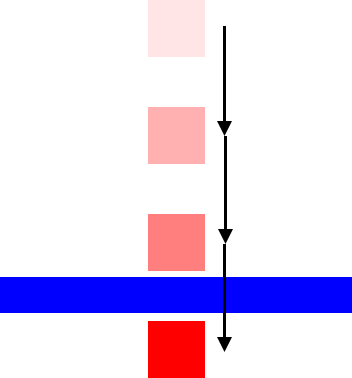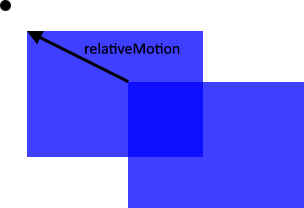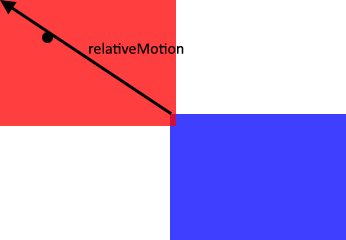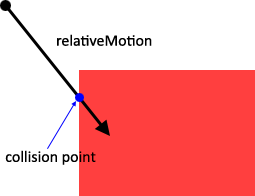# Swept AABB Collision Detection Using the Minkowski Difference (2014-10-05)

Continuing on from yesterday’s post where I explored detecting discrete collisions using Minkowski differences, today I’m going to talk about detecting continuous collisions using Minkowski differences (again, focusing solely on axis-aligned bounding boxes). Continuous collision detection is essential in any game where you have fast-moving objects and/or low frame rates. It adds slightly more complexity to the discrete collision detection algorithm, but the advantages far outweigh the costs in this case!

If you aren’t already familiar with performing discrete collision detection using Minkowski differences, I suggest you read up on that now—what I’m talking about here is an extension of that work. Probably the biggest reason to use swept/continuous collision detection rather than discrete detection is to prevent what is called tunneling, which is shown below.Since we're only solving physics at discrete time points, if an object is moving fast enough (or if the time between discrete points is large enough), said object will jump right "through" an obstacle without a collision ever being detected.

The first thing we need to know about doing continuous collision detection using Minkowski differences is that the technique doesn’t work if the objects are already colliding (i.e., the resultant Minkowski AABB contains the origin). This isn’t too bad however, as if the origin is in the AABB, we can just do discrete collision detection to push the two objects apart. So if the Minkowski AABB cannot contain the origin, then that means that the Minkowski AABB must be located at some distance from the origin:

We also know that if the Minkowski AABB ever contains the origin, then the two objects are colliding. If we are somehow able to construct a vector to move the Minkowski AABB such that it covers the origin, we know that the two objects will collide. If one of the objects were completely static (i.e., not moving), this vector would be the distance moved during the current frame by the moving box, i.e.:

var movementThisFrame:Vector = boxA.velocity * dt;


However, since in this scenario both AABBs could be moving, we must make use of the relative velocity between the two AABBs. The relative velocity between two objects refers to the difference in their velocities:

In a typical world view, each box has its own velocity and is moving of its own accord:

However, if you were to pretend for a moment that the red box is a car in a featureless black box and you were looking at the blue box, you would see its motion relative to you, as if your car were perfectly still and the blue box was the only thing moving:

Conversely, if you were in the blue box looking at the red one, your perception would be different:

So; relative velocity—simple. But what does it have to do with continuous collision detection? Well, since the Minkowski AABB is the Minkowski difference of our two AABBs, it would make sense that the vector which moves the Minkowski AABB over the origin is the difference (relative velocity) of our two AABB’s velocities:

var relativeMotion:Vector = (boxB.velocity - boxA.velocity) * dt;


However note that we aren’t calculating this vector based on how much we need to move the Minkowski AABB to cover the origin—we’re calculating it based on the current velocities of each of the input boxes. Thus, there is no guarantee that this vector will cause the Minkowski AABB to cover the origin and cause the AABBs to collide. However, if it does, we know that the two objects will collide during this frame! Kinda sneaky, eh?If moving the Minkowski AABB by the relative motion vector doesn't cause it to cover the origin, the objects can't collide during this frame.However, if moving the Minkowski AABB by the relative motion vector does cause it to cover the origin, the objects will collide during this frame!

This is fine and dandy if all we want to do is check whether or not the objects will or won’t collide—but if we want to prevent those objects from colliding, we’re going to have to do things a little bit different. We’ll still use the relative velocity, but this time we’ll change the perspective:

var relativeMotion:Vector = (boxA.velocity - boxB.velocity) * dt;


Now, we can ray-trace this relative motion vector from the origin, and see if it collides with our Minkowski AABB (see below). If it does, we get the same result as before with an added bonus—the point on the ray which first touches the AABB defines the point in time when the two objects will start colliding.If the relativeMotion ray cast from the origin doesn't intersect the AABB, then no collision will occur this frame.If the relativeMotion ray cast from the origin does intersect the AABB, then a collision will occur at the collision point.

Once we have the collision point, all that’s left to do is move the AABBs only as far as that collision point and zero their velocity in the normal direction. Note that this is a lot simpler if the collision point is converted into a fractional component $(h)$ of the relativeMotion vector such that:

We can then take $h$ to move the two boxes only as far as they can physically go (without penetrating each other):

In code, this is easy:

var md:AABB = boxB.minkowskiDifference(boxA);
if (md.min.x <= 0 &&
md.max.x >= 0 &&
md.min.y <= 0 &&
md.max.y >= 0)
{
// normal discrete collision detection / separation code
}
else
{
// calculate the relative motion between the two boxes
var relativeMotion:Vector = (boxA.velocity - boxB.velocity) * dt;

// ray-cast the relativeMotion vector against the Minkowski AABB
var h:Float = md.getRayIntersectionFraction(Vector.zero, relativeMotion);

// check to see if a collision will happen this frame
// getRayIntersectionFraction returns Math.POSITIVE_INFINITY if there is no intersection
if(h < Math.POSITIVE_INFINITY)
{
// yup, there WILL be a collision this frame
// move the boxes appropriately
boxA.center += boxA.velocity * dt * h;
boxB.center += boxB.velocity * dt * h;

// zero the normal component of the velocity
// (project the velocity onto the tangent of the relative velocities
//  and only keep the projected component, tossing the normal component)
var tangent:Vector = relativeMotion.normalized.tangent;
boxA.velocity = Vector.dotProduct(boxA.velocity, tangent) * tangent;
boxB.velocity = Vector.dotProduct(boxB.velocity, tangent) * tangent;
}
else
{
// no intersection, move it along
boxA.center += boxA.velocity * dt;
boxB.center += boxB.velocity * dt;
}
}


We just need a couple more helper functions in our AABB class:

// taken from https://github.com/pgkelley4/line-segments-intersect/blob/master/js/line-segments-intersect.js
// which was adapted from http://stackoverflow.com/questions/563198/how-do-you-detect-where-two-line-segments-intersect
// returns the point where they intersect (if they intersect)
// returns Math.POSITIVE_INFINITY if they don't intersect
private function getRayIntersectionFractionOfFirstRay(originA:Vector, endA:Vector, originB:Vector, endB:Vector):Float
{
var r:Vector = endA - originA;
var s:Vector = endB - originB;

var numerator:Float = (originB - originA) * r;
var denominator:Float = r * s;

if (numerator == 0 && denominator == 0)
{
// the lines are co-linear
// check if they overlap
// todo: calculate intersection point
return Math.POSITIVE_INFINITY;
}
if (denominator == 0)
{
// lines are parallel
return Math.POSITIVE_INFINITY;
}

var u:Float = numerator / denominator;
var t:Float = ((originB - originA) * s) / denominator;
if ((t >= 0) && (t <= 1) && (u >= 0) && (u <= 1))
{
return t;
}
return Math.POSITIVE_INFINITY;
}

public function getRayIntersectionFraction(origin:Vector, direction:Vector):Float
{
var end:Vector = origin + direction;

// for each of the AABB's four edges
// calculate the minimum fraction of "direction"
// in order to find where the ray FIRST intersects
// the AABB (if it ever does)
var minT:Float = getRayIntersectionFractionOfFirstRay(origin, end, new Vector(min.x, min.y), new Vector(min.x, max.y));
var x:Float;
x = getRayIntersectionFractionOfFirstRay(origin, end, new Vector(min.x, max.y), new Vector(max.x, max.y));
if (x < minT)
minT = x;
x = getRayIntersectionFractionOfFirstRay(origin, end, new Vector(max.x, max.y), new Vector(max.x, min.y));
if (x < minT)
minT = x;
x = getRayIntersectionFractionOfFirstRay(origin, end, new Vector(max.x, min.y), new Vector(min.x, min.y));
if (x < minT)
minT = x;

// ok, now we should have found the fractional component along the ray where we collided
return minT;
}


And that’s pretty much it! Not too much more work to ensure that two AABBs always collide no matter how fast each of them is moving! A demo showing this functionality off is shown below:

The full source code for this demo is up on Github. If you have any questions, comments, or concerns, I would love to hear from you! I only learned this method of continuous collision detection today, so there’s bound to be some things I missed.